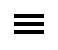Details

# Excel 2013 Formulas

Online Course: ID# 1003598
Qty:
About This Course:
Join LearnKey expert Michael Meskers in our new Excel Formulas course. If you use Microsoft Excel for data analysis, reporting, or day-to-day tracking this course will teach you helpful formulas and hints that will bring ease and accuracy to your work.

Benefits
• Learn time saving formulas that increase accuracy and functionality
• Gain confidence while working in Excel 2013
Session 1
• Section A: Course Introduction
• Basic Formulas
• CONCATENATE
• CONCATENATE, Exponential Formulas
• Text Formulas
• Edit Formulas
• Section B: Convert Formulas to Text
• Split Cell Contents Apart
• Formulas to Text Values
• UPPER, LOWER, PROPER
• LEFT
• RIGHT
• MID
• Fixed with Possibility
• Split Data into Three Columns
• TRIM
• SUBSTITUTE
• REPLACE
• Section C: Basic Numerical Formulas
• AutoSum
• SUM
• AVERAGE, MIN/MAX, COUNT/COUNTA
• Status Bar
• Linked Formulas
• Add Grand Totals
• Data Consolidation
• Section D: AND/OR/NOT
• Working with AND/OR/NOT
• AND
• OR
• NOT
• Subtotal
• Go To
• MEDIAN
• RANK
• Reverse Order RANK
• LARGE
• SMALL
• ISNUMBER
• ISTEXT
• IFERROR
Session 2
• Section A: SUMIF and AVERAGEIFS
• SUMIF
• AVERAGEIF
• COUNTIF
• SUMIFS
• Formula Wizard SUMIFS
• AVERAGEIFS
• COUNTIFS
• IF Formulas
• ABS Formula
• Section B: Nested Formulas
• Working with Nested Formulas
• Calculating Nested Formulas
• Nesting other Formulas inside an IF
• IF(AND
• FIND
• Nest FIND Formula
• INDIRECT Formula
• Column and Row
• Section C: Date Formulas
• DATE Formula
• Calculating DATE Formula
• Weekdays
• NETWORKDAYS
• Working with NETWORKDAYS
• Section D: Conditional Formatting
• New Conditional Formatting Rule
• Conditional Formatting by Formula
• Conflicts in Formatting
• Section E: VLOOKUP
• What is VLOOKUP?
• VLOOKUP Arguments
• VLOOKUP Approximate Match
• VLOOKUP Exact Match
• Testing Exact Match
• Section F: What-If Analysis
• Goal Seek
• Solver
• Solver Parameter
• Scenario Manager
• Input Tables
• Dual Input Tables
• MATCH
• INDEX
• INDEX MATCH
• Section G: Array Formulas
• SUM and MULTIPLICATION
• SUMPRODUCT
• SUBTRACTION and AVERAGE
• TRANSPOSE
Info on how to access this course will be sent to you by the Course Provider via email.
Order:
Excel 2013 Formulas
Price: \$90.00
Qty:
Details
Share This:CPATrainingCenter.com. 5755 North Point Parkway, Suite 227 | Alpharetta, GA 30022 | 770-410-9941 | support@CPATrainingCenter.com
Copyright CPATrainingCenter.com 2018 | Web Site Development by OTAU